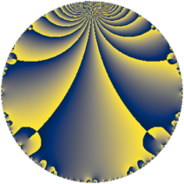Properties

 Label 27.3.dLevel $27$ Weight $3$ Character orbit 27.d Rep. character $\chi_{27}(8,\cdot)$ Character field $\Q(\zeta_{6})$ Dimension $2$ Newform subspaces $1$ Sturm bound $9$ Trace bound $0$

Related objects

Defining parameters

 Level: $$N$$ $$=$$ $$27 = 3^{3}$$ Weight: $$k$$ $$=$$ $$3$$ Character orbit: $$[\chi]$$ $$=$$ 27.d (of order $$6$$ and degree $$2$$) Character conductor: $$\operatorname{cond}(\chi)$$ $$=$$ $$9$$ Character field: $$\Q(\zeta_{6})$$ Newform subspaces: $$1$$ Sturm bound: $$9$$ Trace bound: $$0$$

Dimensions

The following table gives the dimensions of various subspaces of $$M_{3}(27, [\chi])$$.

Total New Old
Modular forms 18 6 12
Cusp forms 6 2 4
Eisenstein series 12 4 8

Trace form

 $$2 q + 3 q^{2} - q^{4} - 6 q^{5} - 2 q^{7} + O(q^{10})$$ $$2 q + 3 q^{2} - q^{4} - 6 q^{5} - 2 q^{7} - 12 q^{10} + 3 q^{11} + 4 q^{13} - 6 q^{14} + 11 q^{16} + 22 q^{19} + 6 q^{20} + 3 q^{22} + 48 q^{23} - 13 q^{25} + 4 q^{28} - 78 q^{29} - 32 q^{31} - 27 q^{32} - 27 q^{34} - 68 q^{37} + 33 q^{38} + 30 q^{40} + 21 q^{41} + 61 q^{43} + 96 q^{46} + 84 q^{47} + 45 q^{49} - 39 q^{50} + 4 q^{52} - 12 q^{55} + 30 q^{56} - 78 q^{58} - 87 q^{59} - 56 q^{61} - 142 q^{64} - 24 q^{65} + 31 q^{67} + 27 q^{68} + 12 q^{70} + 130 q^{73} - 102 q^{74} - 11 q^{76} - 6 q^{77} - 38 q^{79} + 42 q^{82} + 84 q^{83} - 54 q^{85} + 183 q^{86} + 15 q^{88} - 16 q^{91} - 48 q^{92} + 84 q^{94} - 66 q^{95} + 115 q^{97} + O(q^{100})$$

Decomposition of $$S_{3}^{\mathrm{new}}(27, [\chi])$$ into newform subspaces

Label Dim. $$A$$ Field CM Traces $q$-expansion
$a_{2}$ $a_{3}$ $a_{5}$ $a_{7}$
27.3.d.a $2$ $0.736$ $$\Q(\sqrt{-3})$$ None $$3$$ $$0$$ $$-6$$ $$-2$$ $$q+(1+\zeta_{6})q^{2}-\zeta_{6}q^{4}+(-4+2\zeta_{6})q^{5}+\cdots$$

Decomposition of $$S_{3}^{\mathrm{old}}(27, [\chi])$$ into lower level spaces

$$S_{3}^{\mathrm{old}}(27, [\chi]) \cong$$ $$S_{3}^{\mathrm{new}}(9, [\chi])$$$$^{\oplus 2}$$